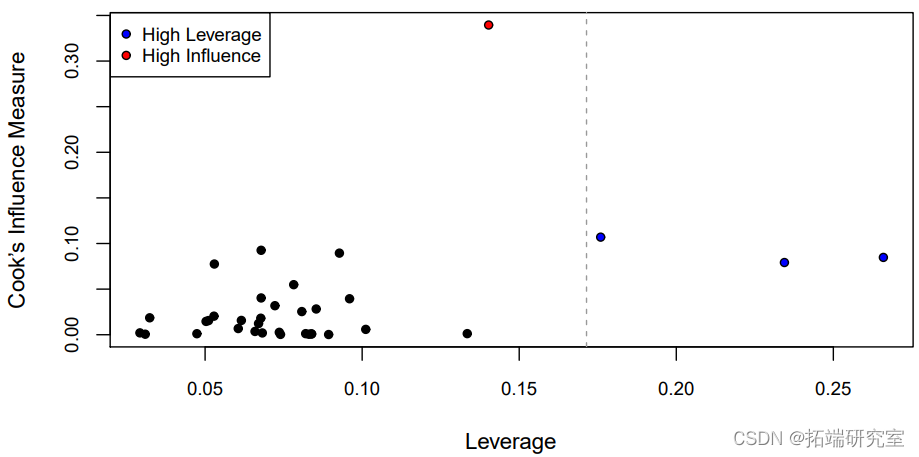# 数据分享|R语言回归模型诊断、离群值分析学生考试成绩、病人医护质量满意度、婴儿死亡率和人均收入、针叶树荫面积数据

•性别：性别男性或女性。

•种族：种族或民族，具有西班牙裔，亚洲人，非洲裔美国人，白人的水平。

•学校类型，公立和私立。

•轨迹：控制位点，一个连续的协变量，指示受试者对影响他们的事件的自我感知控制程度（更高=更感知的控制）

•概念：自我概念，反映受试者自我感知的学术能力的连续协变量（更高=更多的自我感知能力）。

•动机，一个连续的协变量（更高=更多的自我感知的动机）。

•数学：标准化的数学考试成绩。

• 阅读：标准化阅读测试成绩。

`summary(hsb)`

`anova(M1, M2)`

# Matlab中的偏最小二乘法（PLS）回归模型，离群点检测和变量选择

1. 标准化残差：ri = ei σˆ。这些残差是单位的

2. 研究性残差：di = ei σˆ √ 1 – hi

### 随时关注您喜欢的主题

```plot(predict(M1), res, pch = 21, bg = "black", cex = cex, cex.axis = cex,

ex)```

`hist(res/sigma.hat, breaks = 50, freq = FALSE, cex.axis = cex,`

# 线性预测模型

`anova(lm(Satisfaction ~ Age`
`anova(lm(Satisfaction ~ Age, data = satis),`
`anova(lm(Satisfaction ~ Age + Severity, data = satis),`

```pval <- 2*pt(abs(beta.hat)/sqrt(diag(vcov(M))\[-1\]),

df = n-3, lower.tail = FALSE) # pvalues

sim.out\[ii,\] <- round(c(beta.hat\[1\], pval\[1\], beta.hat\[2\], pval\[2\]),2)

}

sim.out```

# 换句话说，βˆ的估计值从一个随机样本到另一个随机样本会有很大的变化。这种现象被称为方差膨胀

```VIF <- diag(solve(cor(X)))

VIF```
```names(satis.star) <- c("Satisfaction", paste0(names(satis)\[-1\], ".star"))

`diag(vcov(M.orig))\["Severity"\]/diag(vcov(M.trans))\["Severity.star"\]`
`VIF\["Severity"\]`

# 离群值

– 收入：以元计的人均收入。

– 婴儿：每1000个活产的婴儿死亡率。

– 地区：大陆，有非洲、美洲、亚洲、欧洲等级别。

– 石油：石油出口国，级别为无、有。

`coef(M) # 系数`
```as.character(formula(M))\[c(2,1,3)\]), collapse = " "), # 模型

xlab = "Predicted Log-Infant Mortality",```

1. 将沙特阿拉伯排除在分析之外，承认我们并不完全知道原因（不推荐）。

2. 将所有9个产油国排除在分析之外，并说明我们的预测只针对非产油国。

3.将沙特阿拉伯排除在外，并评估这对我们的分析有何影响。

# 树木的针叶产生的阴凉面积

```X <- model.matrix(M)

H <- X %*% solve(crossprod(X), t(X)) # HAT matrix

`range(h - diag(H))`

```n <- nrow(lforest)

hbar <- p/n # 平均杠杆率

abline(v = 2*hbar, col = "gray60", lty = 2) # 2x平均杠杆率```其中一个观测值的库克距离几乎是其他观测值的3倍以上（红色），而其中的e个观测值的平均杠杆率是两倍（蓝色）。为了了解为什么这些观测值有很高的杠杆率，我们可以看一下所有协变量的配对图（在这种情况下，这只是高度和树干尺寸）。

```# 用省略的值进行预测

omit.ind <- c(which(inf.ind), # 最有影响力的

which.max(h)) # 最高杠杆率

which(infl.ind)中的错误："which "的参数不符合逻辑

names(omit.ind) <- c("inf", "lev")

omit.ind```
```yobs <- lforest\[, "NeedleArea"\] # 观察值

Mf <- lm(NeedleArea ~ Height + TrunkSize, data = lforest) # 所有观测值

for(jj in 1:length(opt.ind)) {

# 用省略的观察值建立模型

Mo <- lm(NeedleArea ~ Height + TrunkSize,

data = lforest, subset = -omit.ind\[jj\])

yfito <- predict(Mo, newdata = lforest) # 所有观测值的拟合值

for(ii in c("Height", "TrunkSize")) {```

Kaizong Ye拓端研究室（TRL）的研究员。

​非常感谢您阅读本文，如需帮助请联系我们！QQ在线咨询

15121130882

0571-63341498

## 关注有关新文章的微信公众号

This will close in 0 seconds